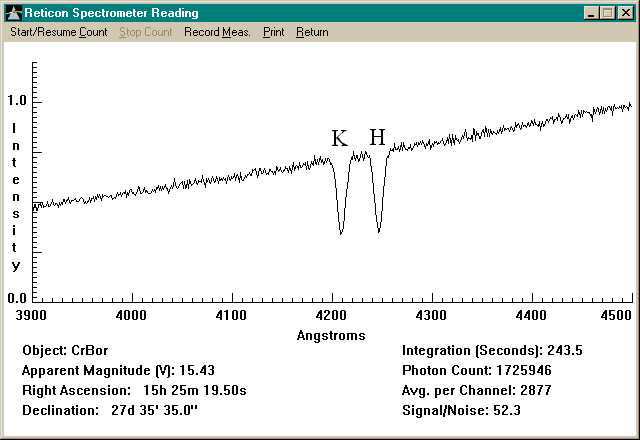On their journey through Intergalactic space, the photons are constantly absorbed and re-emitted, undergoing a shift in wavelength each time. However, as to whether a photon collides or not will depend upon the laws of chance and as a result, some photons will make more collisions than others and thus undergo a different redshift. This means that there will be a spread of redshifts for a particular absorption line. That is, the redshifted absorption lines in the spectra from distant galaxies should not be the sharp lines that we see in the laboratory, but should be 'broadened'. This is what we expect to see and also what we do see.

Let us look at an example and for that example we will take the 'K line' in the spectra produced by ionized calcium. In a stationary object, (non-redshifted) the 'K Line' has a wavelength of 3934 Angstroms or (3.934x10-7 m).This is a print out of the spectrum from Corona Borealis or CorBor galaxy cluster. One can see that the lines have been 'broadened.'

On arrival at the Earth it's wavelength is 4209x10-10 m. The increase in wavelength is 275x10-10 m.

In theight Theory, on each interaction with an electron, the wavelength increases by h/mc

or 2.4x10-12 m each time

(regardless of the initial wavelength).

This means that these photons representing the ‘K’ Line have made
275x10-9 divided by 2.4x10-12 or 11,458 interactions where they were absorbed and re-emitted on the way to us.
Of course this is the mean or average number of collisions, some will make more, some less.

A measure of the ‘spread’ is the ‘standard deviation’ and for this type of data we will take the standard deviation as the square root of the mean number of interactions (a good approximation for large numbers).

Standard Deviation = 107 approx collisions.

Statistics tell us that for data which is distributed normally about the mean, 68% of the data lies within a range of one standard deviation either side of the mean, 95% within a range of two standard deviations either side of the mean and 99.7% should lie within three standard deviations of the mean.

That is 68% of the photons will have made 114,583 +/- 107 collisions.
We can convert this into wavelengths. Each collision increases the wavelength by 2.4x10-12 m
One standard deviation, in wavelength terms is 107x2.4x10-12 m or 2.57x10-10 m.
As a result, the mean redshifted ‘new wavelength’ is 4209x10-10 m.
68% of the photon’s wavelengths will lie in a range (4209 +/- 2.6)x10-10 m
95% of the photon’s wavelengths will lie in a range (4209 +/- 5.2)x10-10 m
99.7% of the photon’s wavelengths will lie in a range (4209 +/- 7.8)x10-10 m.

So 99.7% of our photons will arrive within 7.8x10-10 m either side of the mean value of 4209x10-10 m and this is our spread in the wavelength of the photons representing this absorption line.

Looking at the width of the absorption line shown on the site linked to above, on arrival we see that it is around 15x10-10 m either side of the mean.

Therefore, the experimental spread is more than the statistical spread expected from this New Tired Light Theory.

In other words, the Theory yet again agrees with experiment.

HOME